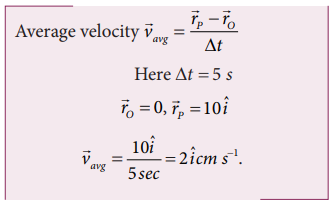Home | | Physics 11th std | Solved Example Problem for Integral Calculus: Average speed, velocity, Momentum

# Solved Example Problem for Integral Calculus: Average speed, velocity, Momentum

Physics : Kinematics : Integral Calculus: Average speed, velocity, Momentum

## Solved Example Problems for Average speed

### Example 2.20

Consider an object travelling in a semi-circular path from point O to point P in 5 second, as is shown in the Figure. Calculate the average velocity and average speed.### SolutionThe average velocity is in the positive x direction.

The average speed = total path length / time taken (the path is semi-circular)Note that the average speed is greater than the magnitude of the average velocity.

## Solved Example Problems Instantaneous velocity or velocity

### Example 2.21

The position vector of a particle is givenCalculate the velocity and speed of the particle at any instant t

Calculate the velocity and speed of the particle at time t = 2 s

SolutionNote that the particle has velocity components along x and y direction. Along the z direction the position has constant value (-5) which is independent of time. Hence there is no z-component for the velocity.

### Example 2.22

The velocity of three particles A, B, C are given below. Which particle travels at the greatest speed?### Solution

We know that speed is the magnitude of the velocity vector. Hence,The particle C has the greatest speed.

### Example 2.23

Two cars are travelling with respective velocities=10ms-1 along east and=10ms-1  along west What  are the speeds of the cars?

### Solution

Both cars have the same magnitude of velocity. This implies that both cars travel at the same speed even though they have velocities in different directions. Speed will not give the direction of motion.## Solved Example Problems Momentum

Example 2.24

Consider two masses of 10 g and 1 kg moving with the same speed 10 m s-1. Calculate the magnitude of the momentum.

Solution

We use p = mv

For the mass of 10 g, m = 0.01 kgThus even though both the masses have the same speed, the momentum of the heavier mass is 100 times greater than that of the lighter mass.

Study Material, Lecturing Notes, Assignment, Reference, Wiki description explanation, brief detail
11th Physics : UNIT 2 : Kinematics : Solved Example Problem for Integral Calculus: Average speed, velocity, Momentum |